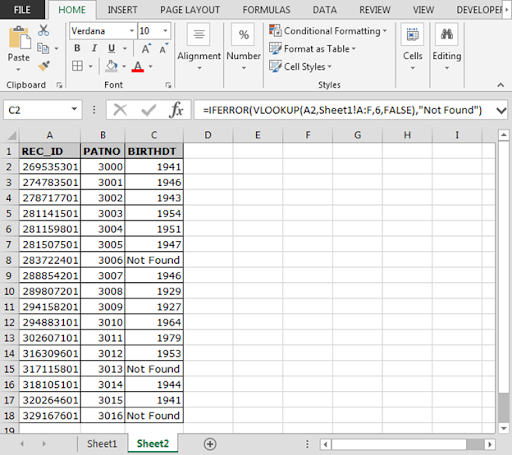# How to use IFERROR with VLOOKUP in Excel?

In this article, we will learn how to use VLOOKUP  IFERROR function to ignore the #N/A error while calculating the formula.

As we all know that Excel IFERROR  function is used to return customised output when an error occurs.

And VLOOKUP  function is used for retrieving the data information in first data to another data on the basis of common value. So when the common value not matched with the second data then formula give us #N/A error as output. we need to tackle if error occurs.

how to use VLOOKUP in Excel?
VLOOKUP in Excel is a function to lookup and retrieves data from a specific column in the table. Lookup values must appear in the first column of the table, with lookup columns to the right. VLOOKUP formula in Excel generates an error if value is not found.

Syntax:

 =VLOOKUP(lookup_value, table_array, col_index_num, [range_lookup])

IFERROR Excel function runs on logic_test. It catches error in a formula and returns an alternative result or formula when an error is detected. #N/A, #VALUE!, #REF!, #DIV/0!, #NUM!, #NAME?, and #NULL!  These errors are checked under IFERROR.

Syntax:

 =IFERROR (value, value_if_error)

Let’s take an example and understand how we can ignore VLOOKUP #N/A Error in Excel.

Here we have two sheets, we have to get the BIRTHDT from SHEET 1 table to SHEET 2 Table using the functions.

Write the IFERROR formula in C2 cell of SHEET 2.

 =IFERROR(VLOOKUP(A2,Sheet1!A:F,6,FALSE),”NotFound”)

Explanation:
The VLOOKUP function looks for the 6th column of the SHEET 1 matching  REC_ID in SHEET 1 from SHEET 2.
IFERROR catches the error in the VLOOKUP formula and returns “Not Found”

Copy the formula in other cells, select the cells taking the first cell where the formula is already applied, use shortcut key Ctrl + DAs you can see in the above image that formula is working fine. And the user can work without getting irritated by #NA error.

what is vlookup used for?
Vlookup function is used to search and  retrieves data from a specific column in the table.

Hope you understood how to use the VLOOKUP function in Excel. You can perform these function in Excel 2016, 2013 and 2010. There are more articles on VLOOKUP and HLOOKUP.  Please do check more links here and if you have any unresolved query, please state that in the comment box below. We will help you.

Popular Articles

The VLOOKUP Function in Excel

COUNTIF in Excel 2016

How to Use SUMIF Function in Excel

1.